# I would like to get the energy spectrum of β particles in a proportional counting tube, do you have any suggestions?

I tried modifying the Geant4GarfieldInterface in it, but each event took too long to calculate and the following issue occurred，I changed the gas file and kept the material in geant4 consistent,
1，is there a way to reduce the calculation time（Each event takes tens of seconds to calculate） and get the energy spectrum of the particles in the proportional counting tube；

2，When the emitted particle energy is greater than 200 keV, it is not completely deposited in the tube, is this normal?

What gas gain do you expect in your proportional counter? If the gain is large, the avalanche simulation using `AvalancheMicroscopic` may take a long time; you could try using `DriftLineRKF` instead.

I don’t currently care about gas gain, I want to get the energy spectrum of particles in proportional counter，Is there an example of the use of DriftLineRK?

“MediumMagboltz::GetElectronCollisionRate” appears:
Rate at 40.2529 eV is not included in the current table" does it affect the accuracy of the calculation?

No, it just means that the table of collision rates is recomputed.

This example is probably a good starting point:

https://garfieldpp.web.cern.ch/garfieldpp/examples/tube/

In the Geant4GarfieldInterface example, whether the emitted particles (not electron ion pairs) are affected by the electric field corresponding to the gasfile

Thank you, I’ll have a look at this example carefully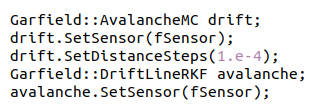hi，I try using `DriftLineRKF` instead，but there are some errors 。
When I comment this line，Calculations still take a long time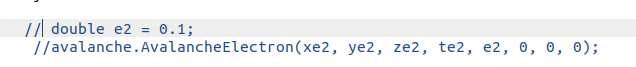`DriftLineRKF` does not have a function `AvalancheElectron`; the function you need called is `DriftElectron`. In fact, if you are using `DriftLineRKF` there is no need to use `AvalancheMC` to first drift the electron to the wire. You can call `DriftLineRKF::DriftElectron` using the starting point of the electron.

hi，I am now using DriftLineRKF as shown in the image below, but the gas energy deposition does not seem to be quite right, I don’t know what is the reason for the initial decline,If I set which parameter is wrong，i use “e-” with 70 keV energy.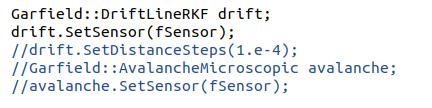Another problem is that I use this formula to calculate the density of the gas mixture, which is not equal to the density in the program，not equal to 1.8223.I wonder if it is calculated with another formula.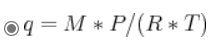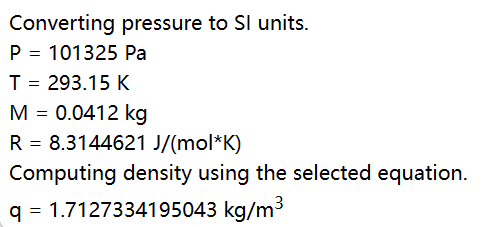Hi,
sorry for the late reply. The mixture ratio 70:30 usually refers to the mole fraction (or volume fraction since we assume it’s an ideal gas), not the mass fraction.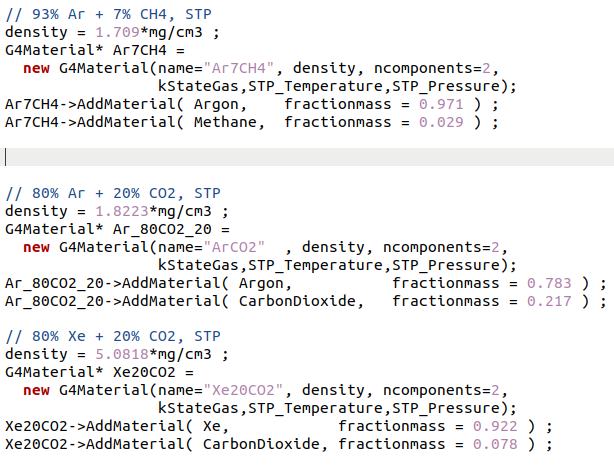I found this in TestEm8, which I used in the mixed gas calculation above, using the molar mass ratio.

This topic was automatically closed 14 days after the last reply. New replies are no longer allowed.